### 2、dp vs 百分比

• dp

Density-independent pixel (dp)獨立畫素密度。標準是160dip.即1dp對應1個pixel，計算公式如：px = dp * (dpi / 160)，螢幕密度越大，1dp對應 的畫素點越多。

• 呈現效果仍舊會有差異，僅僅是相近而已
• 當裝置的物理尺寸存在差異的時候，dp就顯得無能為力了。為4.3寸螢幕準備的UI，執行在5.0寸的螢幕上，很可能在右側和下側存在大量的空白。而5.0寸的UI執行到4.3寸的裝置上，很可能顯示不下。

``````<?xml version="1.0" encoding="utf-8"?>
<resources>
<!-- values-hdpi 480X800 -->
<dimen name="imagewidth">120dip</dimen>
</resources>

<resources>
<!-- values-hdpi-1280x800 -->
<dimen name="imagewidth">220dip</dimen>
</resources>

<?xml version="1.0" encoding="utf-8"?>
<resources>
<!-- values-hdpi  480X320 -->
<dimen name="imagewidth">80dip</dimen>
</resources> ``````

• 百分比
這個概念不用說了，web中支援控制元件的寬度可以去參考父控制元件的寬度去設定百分比，最外層控制元件的寬度參考螢幕尺寸設定百分比，那麼其實中Android裝置中，只需要支援控制元件能夠參考螢幕的百分比去計算寬高就足夠了。

• 對於圖片展示的Banner，為了起到該有的效果，我希望在任何手機上顯示的高度為螢幕高度的1/4
• 我的首頁分上下兩欄，我希望每個欄目的螢幕高度為11/24，中間間隔為1/12

``````   <Button
android:text="@string/hello_world"
android:layout_width="20%w"
android:layout_height="10%h"/>``````

• some 適配tips

1. 多用match_parent
2. 多用weight
3. 自定義view解決

### 3、百分比的引入

#### 1、引入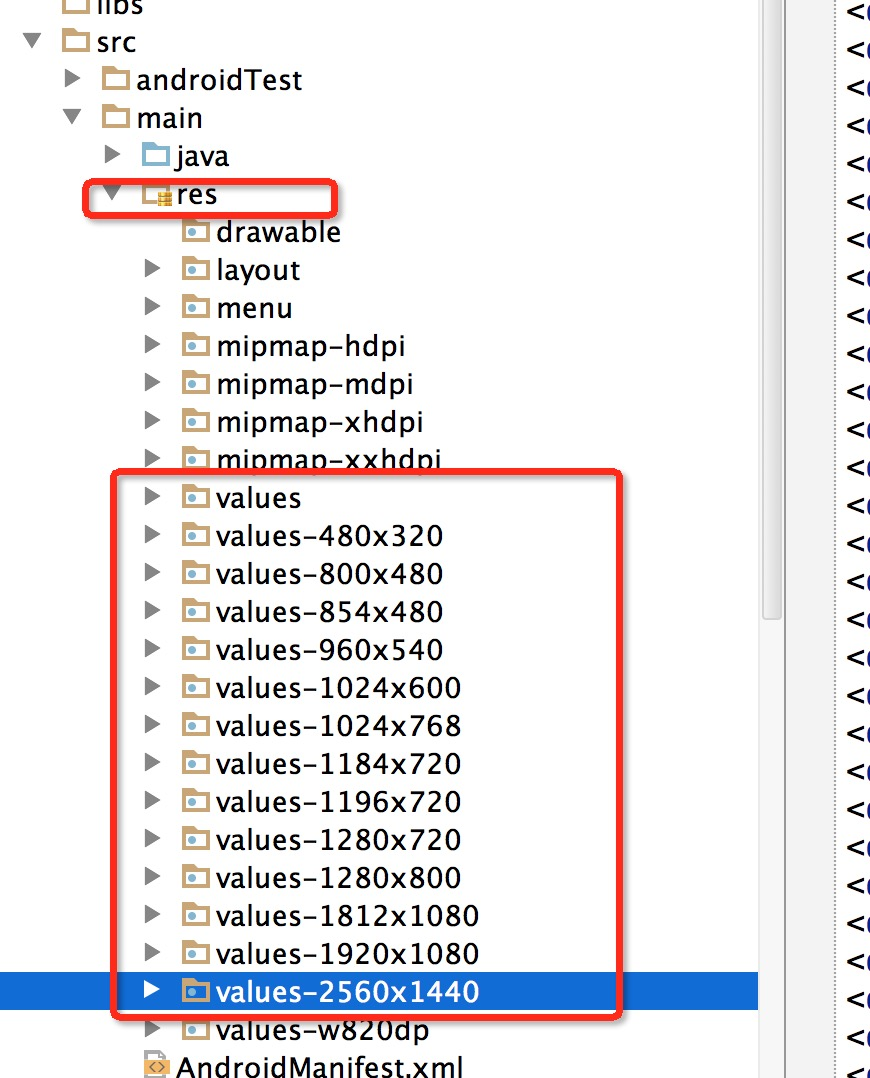• 寬度為320，將任何解析度的寬度分為320份，取值為x1-x320
• 高度為480，將任何解析度的高度分為480份，取值為y1-y480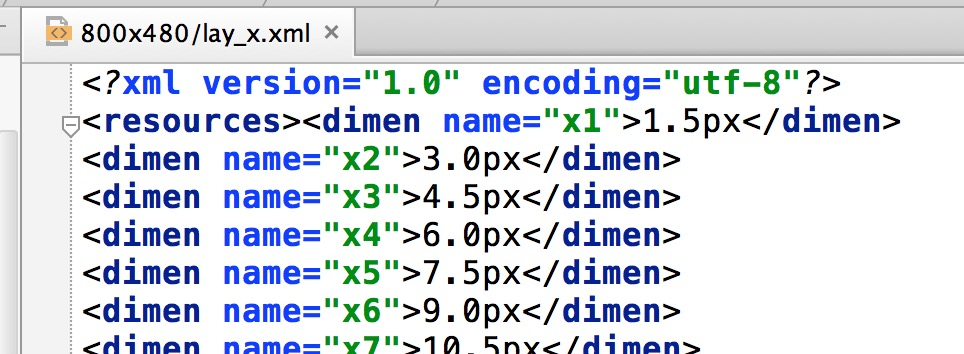``````<FrameLayout >

<Button
android:layout_gravity="center"
android:gravity="center"
android:text="@string/hello_world"
android:layout_width="@dimen/x160"
android:layout_height="@dimen/x160"/>

</FrameLayout>``````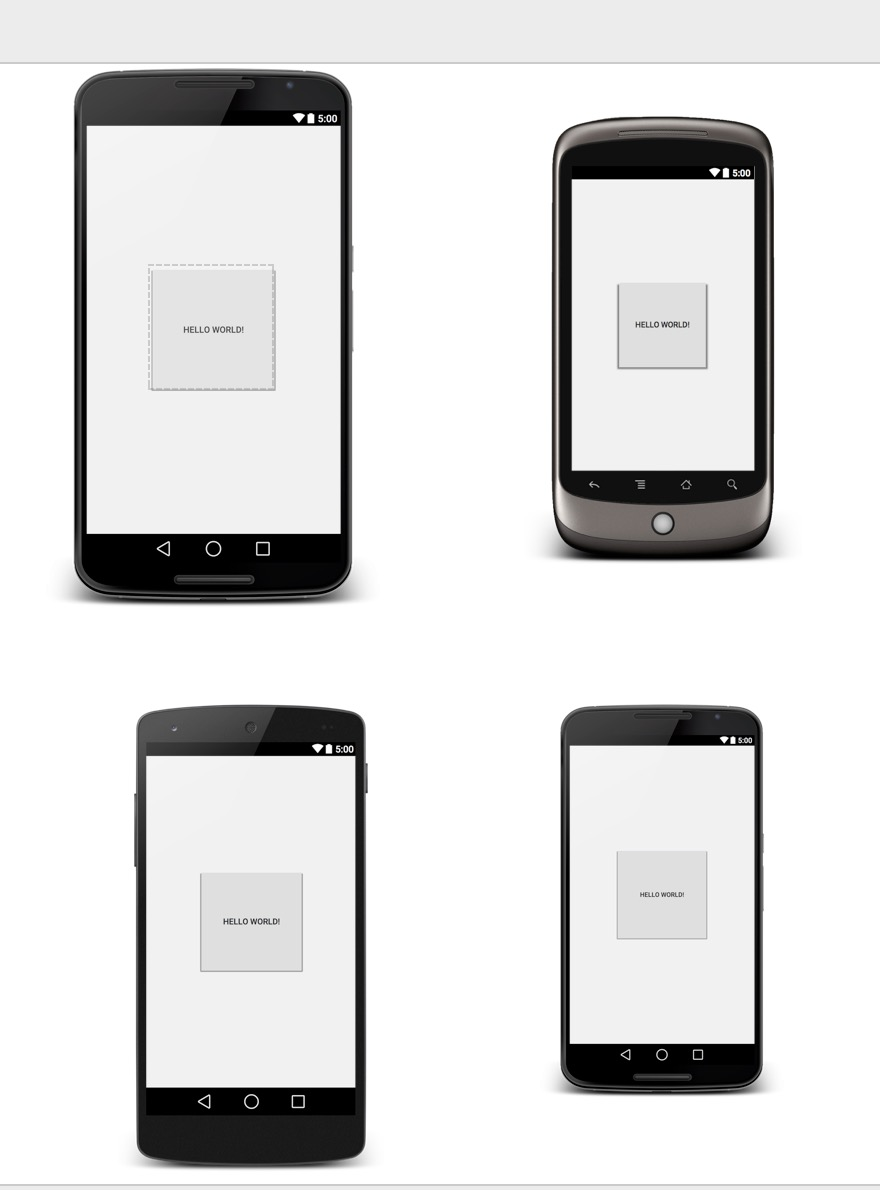• 對於設計圖

• 對於上文提出的幾個dp做不到的

#### 2、自動生成工具

1. 分析需要的支援的解析度

1. 編寫自動生成檔案的程式

``````import java.io.File;
import java.io.FileNotFoundException;
import java.io.FileOutputStream;
import java.io.PrintWriter;

/**
* Created by zhy on 15/5/3.
*/
public class GenerateValueFiles {

private int baseW;
private int baseH;

private String dirStr = "./res";

private final static String WTemplate = "<dimen name=\"x{0}\">{1}px</dimen>\n";
private final static String HTemplate = "<dimen name=\"y{0}\">{1}px</dimen>\n";

/**
* {0}-HEIGHT
*/
private final static String VALUE_TEMPLATE = "values-{0}x{1}";

private static final String SUPPORT_DIMESION = "320,480;480,800;480,854;540,960;600,1024;720,1184;720,1196;720,1280;768,1024;800,1280;1080,1812;1080,1920;1440,2560;";

private String supportStr = SUPPORT_DIMESION;

public GenerateValueFiles(int baseX, int baseY, String supportStr) {
this.baseW = baseX;
this.baseH = baseY;

if (!this.supportStr.contains(baseX + "," + baseY)) {
this.supportStr += baseX + "," + baseY + ";";
}

this.supportStr += validateInput(supportStr);

System.out.println(supportStr);

File dir = new File(dirStr);
if (!dir.exists()) {
dir.mkdir();

}
System.out.println(dir.getAbsoluteFile());

}

/**
* @param supportStr
*            w,h_...w,h;
* @return
*/
private String validateInput(String supportStr) {
StringBuffer sb = new StringBuffer();
String[] vals = supportStr.split("_");
int w = -1;
int h = -1;
String[] wh;
for (String val : vals) {
try {
if (val == null || val.trim().length() == 0)
continue;

wh = val.split(",");
w = Integer.parseInt(wh);
h = Integer.parseInt(wh);
} catch (Exception e) {
System.out.println("skip invalidate params : w,h = " + val);
continue;
}
sb.append(w + "," + h + ";");
}

return sb.toString();
}

public void generate() {
String[] vals = supportStr.split(";");
for (String val : vals) {
String[] wh = val.split(",");
generateXmlFile(Integer.parseInt(wh), Integer.parseInt(wh));
}

}

private void generateXmlFile(int w, int h) {

StringBuffer sbForWidth = new StringBuffer();
sbForWidth.append("<?xml version=\"1.0\" encoding=\"utf-8\"?>\n");
sbForWidth.append("<resources>");
float cellw = w * 1.0f / baseW;

System.out.println("width : " + w + "," + baseW + "," + cellw);
for (int i = 1; i < baseW; i++) {
sbForWidth.append(WTemplate.replace("{0}", i + "").replace("{1}",
change(cellw * i) + ""));
}
sbForWidth.append(WTemplate.replace("{0}", baseW + "").replace("{1}",
w + ""));
sbForWidth.append("</resources>");

StringBuffer sbForHeight = new StringBuffer();
sbForHeight.append("<?xml version=\"1.0\" encoding=\"utf-8\"?>\n");
sbForHeight.append("<resources>");
float cellh = h *1.0f/ baseH;
System.out.println("height : "+ h + "," + baseH + "," + cellh);
for (int i = 1; i < baseH; i++) {
sbForHeight.append(HTemplate.replace("{0}", i + "").replace("{1}",
change(cellh * i) + ""));
}
sbForHeight.append(HTemplate.replace("{0}", baseH + "").replace("{1}",
h + ""));
sbForHeight.append("</resources>");

File fileDir = new File(dirStr + File.separator
+ VALUE_TEMPLATE.replace("{0}", h + "")//
.replace("{1}", w + ""));
fileDir.mkdir();

File layxFile = new File(fileDir.getAbsolutePath(), "lay_x.xml");
File layyFile = new File(fileDir.getAbsolutePath(), "lay_y.xml");
try {
PrintWriter pw = new PrintWriter(new FileOutputStream(layxFile));
pw.print(sbForWidth.toString());
pw.close();
pw = new PrintWriter(new FileOutputStream(layyFile));
pw.print(sbForHeight.toString());
pw.close();
} catch (FileNotFoundException e) {
e.printStackTrace();
}
}

public static float change(float a) {
int temp = (int) (a * 100);
return temp / 100f;
}

public static void main(String[] args) {
int baseW = 320;
int baseH = 400;
try {
if (args.length >= 3) {
baseW = Integer.parseInt(args);
baseH = Integer.parseInt(args);
} else if (args.length >= 2) {
baseW = Integer.parseInt(args);
baseH = Integer.parseInt(args);
} else if (args.length >= 1) {
}
} catch (NumberFormatException e) {

System.err
.println("right input params : java -jar xxx.jar width height w,h_w,h_..._w,h;");
e.printStackTrace();
System.exit(-1);
}

}

}``````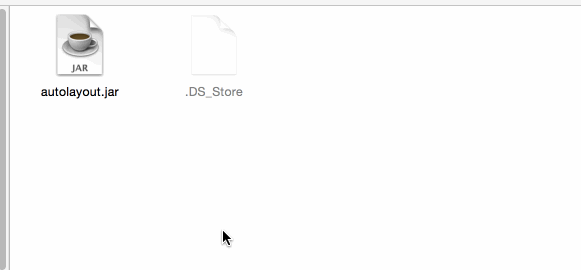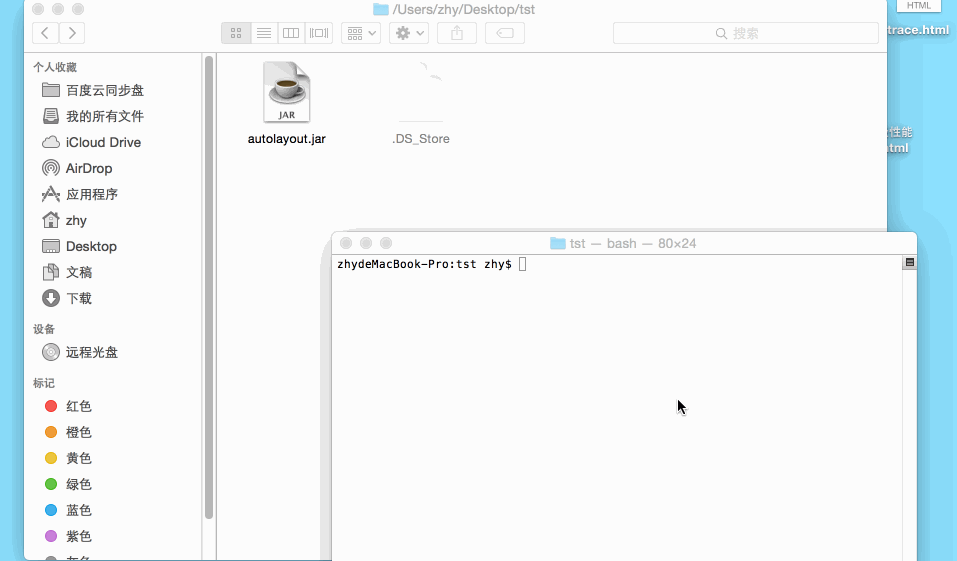java -jar xx.jar width height width,height_width,height

===>後期更新

ok~